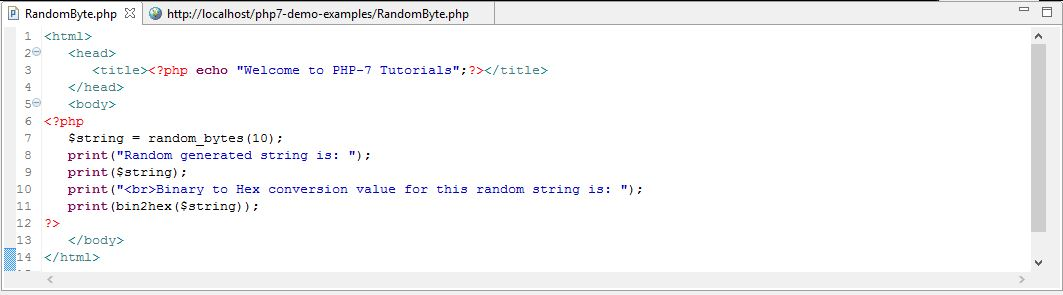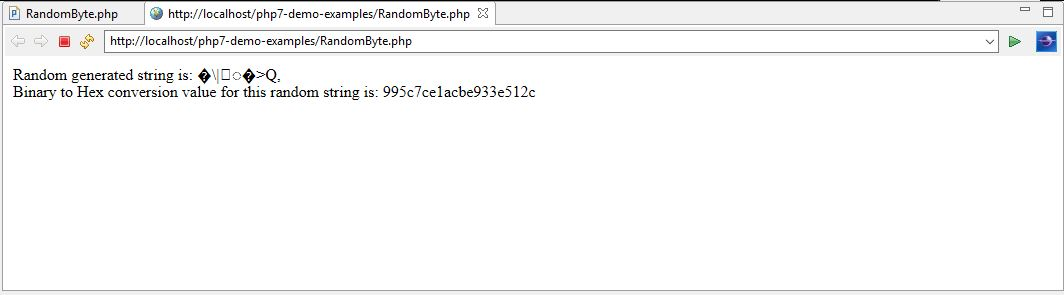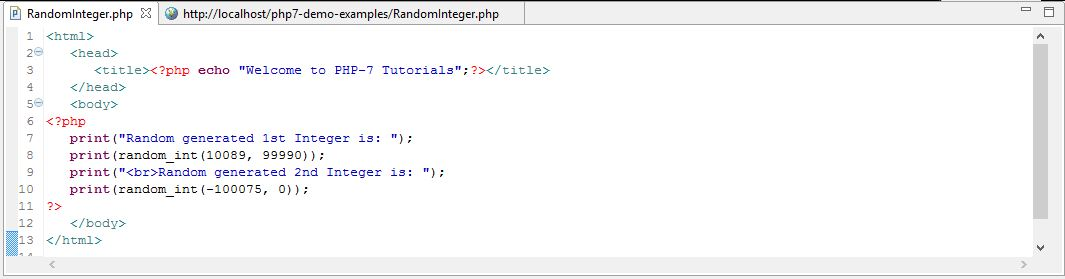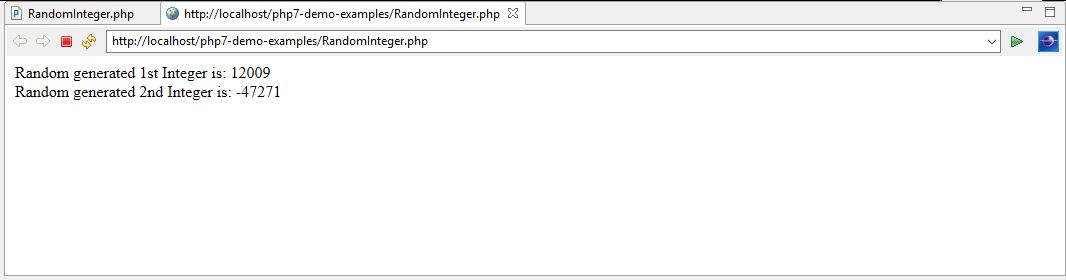Web Programming TutorialsLearn How to Use CSPRNG in PHP 7

# Learn How to Use CSPRNG in PHP 7In the last chapter, we discussed about ‘IntlChar’ class with examples. In this chapter, we are going to discuss about ‘CSPRNG’ that is added to PHP 7.  ‘CSPRNG’ functions are basically used to generate random strings and integers.

Application of random strings and Integers
Generated random numbers and strings by the computer program have many uses in the field of cryptography, science, art, statistics, gambling, gaming, etc. For example, in lottery systems the wining number is a random number generated by the computer program which may turn a person into a millionaire overnight. When we register our phone number on a particular website, then that website authenticates our phone by sending a random number via SMS that we enter on that website to complete the authentication process or we receive a temporary password in case of forget password which is nothing but just a random string or an integer. These random numbers or strings, somewhere in the background are generated by the computer programs. Let’s understand these ‘CSPRNG’ functions in PHP 7.

CSPRNG
CSPRNG stands for ‘Cryptographically Secure Pseudo-Random Number Generator’. In cryptography, we require random numbers, in the case of key generation, nonce, one-time pads, salts in certain signature schemes, etc. Hence, the CSPRNG is named after this phenomenon.

PHP 7 includes two new functions which are included to generate cryptographically secure pseudo-random integers and strings in a cross-platform method. These functions are as follows.

• random_bytes () – This function is used to generate an arbitrary-length string of cryptographically secure pseudo-random bytes. These bytes has only cryptographic use like when we generate keys or initialize vectors as discussed before. Following is the syntax for this function.

Syntax
string random_bytes ( int \$length );

• Parameter – The function accepts the length of the string as an input parameter. It is of integer type and given in terms of number of bytes.
• Return Value – The function returns a string value which is cryptographically secure random bytes.
• Errors/Exceptions – Exceptions or errors will be thrown by the function in the following scenarios.
• If no source of randomness is found then an exception will be thrown.
• A TypeError will be thrown when input parameters are invalid.
• An error will be thrown when input parameter is passed which has an invalid length.

Example
In the below example, we are going to generate a random string of length 10 bytes.Explanation of code

• Following the syntax of the ‘random_bytes’ CSPRNG function, we are generating a string of cryptographically secure pseudo-random bytes.
• Since the input parameter is passed as 10. Therefore, system will generate a random string of length 10 bytes. This string is returned by the function.
• Next we are converting the binary code into the hexadecimal code for the random generated string by using the in-built function ‘bin2hex’.

Output
As explained above, the system first generates a random string of length 10 bytes and then converts the string’s binary code in hexadecimal code as shown below.• random_int () – This function is used to generate cryptographically secure pseudo-random integers. These integers are only used when the unbiased results are critical. Following is the syntax for this function.

Syntax
int random_int ( int \$min , int \$max );

• Parameters – The function accepts two input parameters ‘\$min’ and ‘\$max’. Both of these parameters accept integer values.

As the name suggests ‘\$min’ is the lowest value that is to be returned which could be either PHP_INT_MIN or higher.

On the other hand ‘\$max’ is the highest value that to be returned which could be, either less than or equal to PHP_INT_MAX.

• Return Value – The function returns an integer value which is a cryptographically secure random integer. This integer value will always be between the min and max range that were given as input parameters.
• Errors/Exceptions – Exception or error will be thrown by the function in the following scenarios.
• If no source of randomness is found then an exception will be thrown.
• A TypeError will be thrown when input parameters are invalid.
• An error will be thrown when the value of ‘\$max’ is given less than the value of ‘\$min’ as input parameters.

Example
In the below example, we are going to generate a random integer between pre-defined minimum and maximum range.Explanation of code

• Following the syntax of the ‘random_int’ CSPRNG function, we are generating an integer number of cryptographically secure pseudo-random bytes.
• Since the input parameters in the first case are passed as ‘10089’ and ‘99990’. Therefore, system will generate a random integer between range (10089, 99990). This will be a positive number. This integer number is returned by the function.
• Since the input parameters in the second case are passed as ‘-100075’ and zero. Therefore, system will generate a random integer between range (-100075, 0). This will be a negative number. This integer number is returned by the function.

Output
As explained above, here the system first generates a random integer number between range (10089, 99990) i.e. a positive number then it generates a negative number that is between range (-100075, 0) as shown below.Conclusion
In this chapter, we explored ‘CSPRNG’ (Cryptographically Secure Pseudo-Random Number Generator’) that is added to PHP 7 as oppose to PHP 5.x. In the coming chapters of this tutorial, we are going to discuss about exceptions and errors handling that have added to PHP7.

### How SEO Impacts Your Sales

21,501Fans
4,209Followers
104,000Subscribers

### How SEO Impacts Your Sales

- Advertisement -